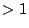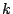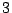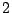# Clique Coloring of Perfect Graphs

It has been asked by Duffus et al [ MR 92e:06009] whether the clique hypergraph of perfect graphs is colorable with a constant number of colors. Several results followed showing that for some classes of perfect graphs this constant is actually 2 or 3 :

They proved it for comparability and cocomparability graphs, Bacsó et al proved the same for some more perfect graphs, and noticed that 'almost all' perfect graphs are 3-clique-colorable (applying Prömel and Steger's result (Probability and Computation, 1, 1992)); they ask whether this bound holds for all perfect graphs:

Can the vertex-set of any perfect graph be partitioned into three classes, so that no (inclusionwise) maximal clique (of size) is included in any of these ? Is the same true already for odd hole free graphs ?

If the clique-hypergraph of a graph and of all of its subgraphs can be colored withcolors, that is, there exists a partition of the vertex-set intoparts so that none of them contains an (inclusionwise) maximal clique, then Hoàng and McDiarmid [ MR 2002j:05110] say the graph is strongly-divisible. This is indeed a sharpening of-divisibility where `maximal' is replaced by `maximum' (cardinality). In these terms the above conjecture states that perfect graphs are strongly-divisible. We formulate another problem in this language:

Can strong-divisibility be decided in polytime ?

A major difficulty with the coloration of the maximal clique hypergraph is that it is NP-hard to decide whether a partition of the vertices is a clique-coloration, and even in very particular classes of perfect graphs.

Contributed by Myriam Preissmann and András Sebo

Back to the main index for Perfect Graphs.